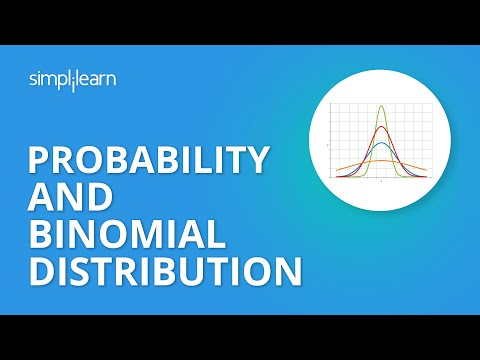Tutorial PlaylistProbability and statistics have enormous applications in data science, with artificial intelligence and machine learning relying heavily on them. In this article, you will learn Binomial Distribution, an essential part of the probability function.

What Is a Random Variable?

A random variable is a variable whose value is not known. It can either be discrete (having a specific value) or continuous (any value in a continuous range). All possible values that a random variable accepts is also called a sample space.

Post Graduate Program in Data Analytics

In partnership with Purdue UniversityWhat Is a Binomial Random Variable?

A binomial random variable is a number of successes in an experiment consisting of N trails. Some of the examples are:

• The number of successes (tails) in an experiment of 100 trials of tossing a coin. Here the sample space is {0, 1, 2, …100}
• The number of successes (four) in an experiment of 100 trials of rolling a dice. Here the sample space is {0, 1, 2, …100}

What Is Binomial Distribution?

In a binomial experiment, the binomial distribution is a discrete probability distribution that represents the probabilities of binomial random variables. The binomial distribution is a probability distribution associated with a binomial experiment in which the binomial random variable specifies the number of successes or failures that occurred within that sample space.

Let’s take an example. Suppose you flipped a coin. The probability of getting heads or tails is equal. But what will be the probability of getting six heads in ten flips of coins? This is where you will need binomial distribution. You can calculate the probability of getting six heads in ten flips of a coin.

The binomial distribution formula for any random variable X is given by

P(x, n, P) = nCx * Px * (1 - P)n-x

Where,

n = the number of experiments

x = 0, 1, 2, 3, 4, … (total number of successes)

p = Probability of success in a single experiment

Let’s calculate the probability of getting exactly six heads when a coin is tossed ten times.

We will use the formula, P(x, n, P) = nCx * Px * (1 - P)n-x

where n = 10, p = 0.5, x = 6

P(x=6) = 10C6 * 0.56 * 0.54 = 0.205

FREE Course: Introduction to Data Analytics

Learn Data Analytics Concepts, Tools & SkillsMean and Variance of Binomial Distribution

The mean and variance of the binomial distribution are:

Mean = np

Variance = npq

where,

p is the probability of success

q is the probability of failure (1-p)

n is the number of trials.

Properties of Binomial Distribution

The properties of a binomial distribution are:

1. There are only two possible outcomes: True or False, Yes or No.
2. There are N number of independent trials.
3. The probability of success and failure varies in each trial.
4. Only the number of successes are taken into account out of N independent trials.

Binomial Distribution Python: Example

Let’s analyze the following python example to get an idea of the binomial distribution.• Here N is the number of trials, and p is the probability of getting success in each trial.
• The binomial random variable, X, shows the number of successes in each experiment.

The output plot that you will get after executing the code is shown below.If you consider the above graph, the probability of getting success is 0.175.

Looking forward to a career in Data Analytics? Check out the Data Analytics Cetification Training and get certified today.

Conclusion

In this “Understanding the Fundamentals of Binomial Distribution“ article, you have learned about Binomial Distribution and its properties along with an example to better understand the concept.

If you are keen on learning about Binomial Distribution and related statistical concepts, you could explore a career in data analytics. Simplilearn’s Post Graduate Program in Data Analytics is one of the most comprehensive online programs for Data Analytics.

If you have any questions or queries related to this tutorial or our certification course, let us know in the comments section of this article, and our experts will be happy to answer.Simplilearn